International
Tables for
Crystallography
Volume D
Physical properties of crystals
Edited by A. Authier

International Tables for Crystallography (2006). Vol. D, ch. 1.1, pp. 32-33

## Section 1.1.6. Glossary

A. Authiera*

aInstitut de Minéralogie et de la Physique des Milieux Condensés, Bâtiment 7, 140 rue de Lourmel, 75015 Paris, France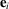basis vectors in direct space (covariant)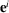basis vectors in reciprocal space (contravariant)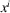components of a vector in direct space (contravariant)components of a vector in reciprocal space (covariant)components of the metric tensor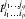components of a tensor of rank n, p times covariant and q times contravariant (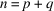)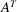transpose of matrix Atensor product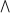outer product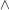vector product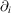partial derivative with respect to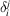Kronecker symbolpermutation tensor V volume p pressurecomponents of the displacement vectorcomponents of the strain tensor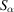components of the strain Voigt matrixcomponents of the stress tensor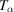components of the stress Voigt matrix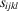elastic compliances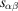reduced elastic compliances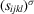adiabatic elastic complianceselastic stiffnesses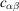reduced elastic stiffnesses ν Poisson's ratio E Young's modulus Θ temperature σ entropythermal expansiontemperature-stress constantinternal energyGibbs free energy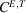specific heat at constant stress and applied electric field E electric field D electric displacement H magnetic field B magnetic induction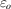permittivity of vacuumdielectric constant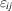dielectric tensor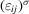adiabatic dielectric tensor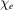dielectric susceptibility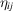dielectric impermeabilitypyroelectric tensor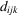piezoelectric tensor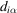reduced piezoelectric tensor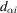reduced inverse piezoelectric tensor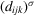adiabatic piezoelectric tensorpiezoelectric tensor at constant strain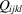electrostriction tensor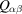reduced electrostriction tensor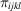piezo-optic tensor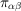reduced piezo-optic tensor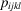elasto-optic tensor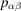reduced elasto-optic tensor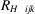Hall constant